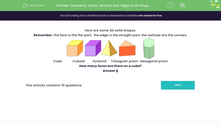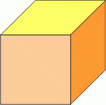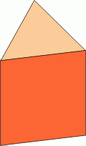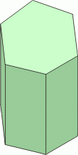# Geometry: Faces, Vertices and Edges of 3D Shapes

In this worksheet, students answer questions on the number of faces, edges and vertices on some 3D shapes.Key stage:  KS 2

Curriculum topic:   Geometry: Properties of Shapes

Curriculum subtopic:   Know 3D Shapes

Difficulty level:#### Worksheet Overview

Here are some 3D solid shapes.

Remember, the face is the flat part,  the edge is the straight part, the vertices are the corners.Cube            Cuboid         Pyramid     Triangular prism  Hexagonal prism

How many faces are there on a cube?